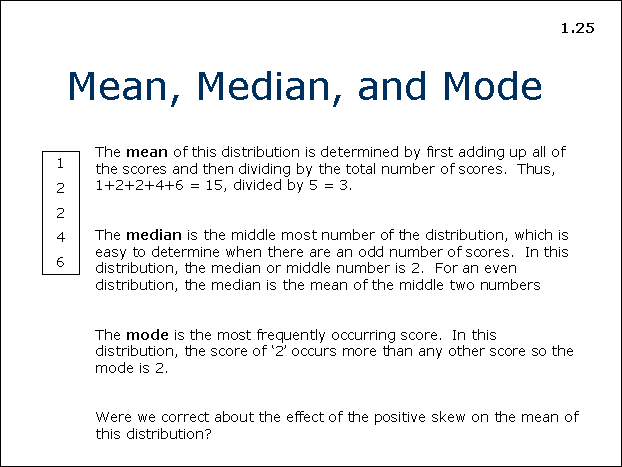# 25: Mean, Median, and Mode## Mean, Median, and Mode

The mean of this distribution is determined by first adding up all the scores and then dividing by the total number of scores. Thus, 1+2+2+4+6 = 15, divided by 5 = 3.

The median is the middle most number of the distribution, which is easy to determine when there are an odd number of scores. In this distribution, the median or middle number is 2. For an even distribution, the median is the mean of the two middle numbers.

The mode is the most frequently occurring score. In this distribution, the score of ‘2’ occurs more than any other score so the mode is 2.

Were we correct about the effect of the positive skew on the mean of the distribution?